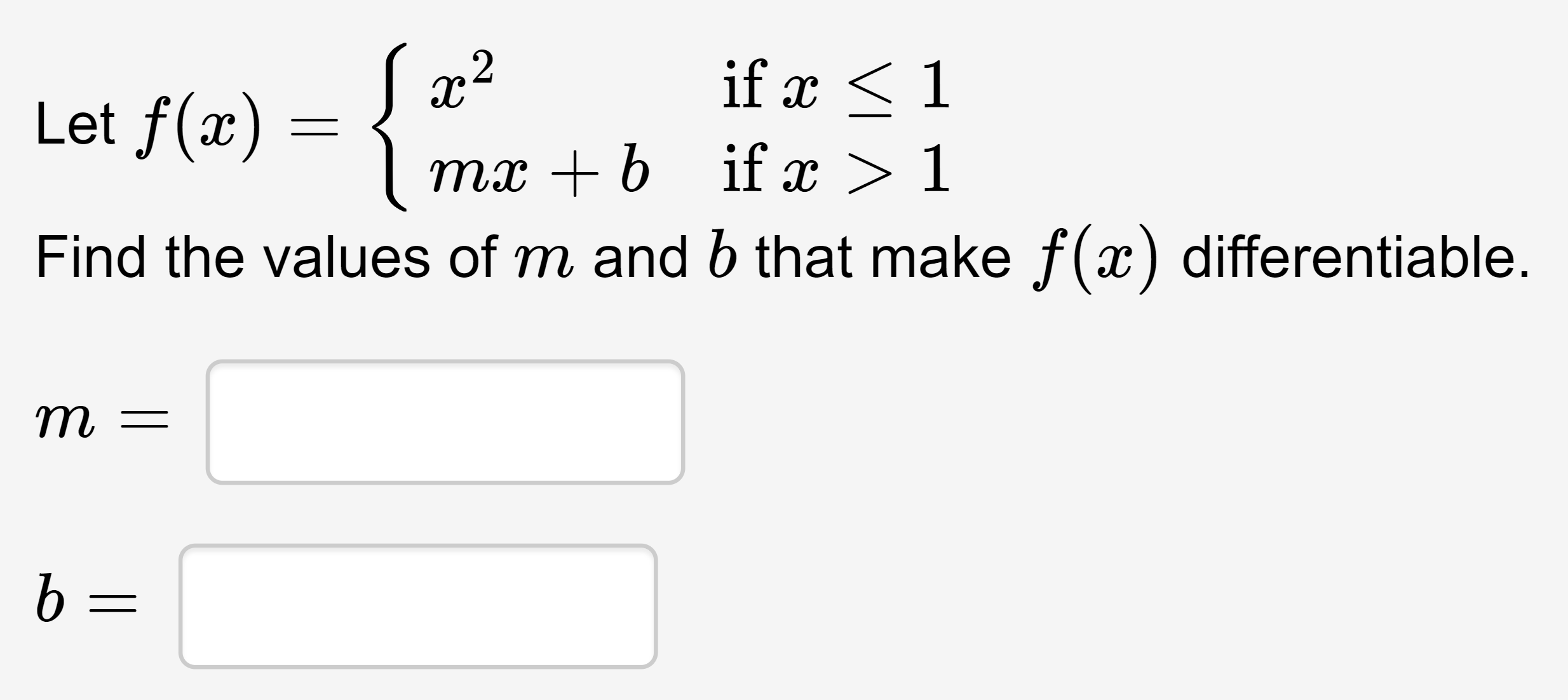# if x1Let f(x)mx b if x > 1Find the values of m and 6 that make f(x) differentiable.т

Question
3 viewshelp_outlineImage Transcriptioncloseif x1 Let f(x) mx b if x > 1 Find the values of m and 6 that make f(x) differentiable. т fullscreen
check_circle

star
star
star
star
star
1 Rating
Step 1

Given :

Step 2

Theorem: A function f(x) is differentiable if and only if f’(x) is continuous.

Step 3

Using above theorem differentiating the given functi...

### Want to see the full answer?

See Solution

#### Want to see this answer and more?

Solutions are written by subject experts who are available 24/7. Questions are typically answered within 1 hour.*

See Solution
*Response times may vary by subject and question.
Tagged in

### Continuity